# Design of a New Duty Cycle Modulation to Improve the Energy Quality of an Insulated Production System

Design of a New Duty Cycle Modulation to Improve the Energy Quality of an Insulated Production System

Clément Kengnou Donfack Charles Hubert KomJean Jacques Mandeng Félix Paune

Laboratory of Computer Engineering and Automation, Higher Normal School of Technical Education of Douala, University of Douala, Po Box 2701 Douala, Cameroon

Laboratory of Energy, Materials, Modelling and Methods, National Higher Polytechnic School of Douala, University of Douala, PO Box 2701 Douala, Cameroon

Corresponding Author Email:
charleshubert.kom@gmail.com
Page:
311-319
|
DOI:
https://doi.org/10.18280/ejee.230404
8 October 2020
|
Accepted:
18 May 2021
|
Published:
31 August 2021
| Citation

OPEN ACCESS

Abstract:

In this article, we propose a new strategy for controlling three-phase inverters of renewable energy sources, based on the Duty Cycle Modulatiom (DCM) control using the Park and Fortescue transformation (DCM-dq-dih). Our goals in setting and using this strategy are, on the one hand, to induce a lower harmonic rate as compared to the SPWM (Sinusoidal Pulse Width Modulation) strategy and on the other hand, this control technique enables the inverter to deliver balanced voltages, be it in the event of load unbalance. Our design is built on the basis of the known mode of DCM control of single-phase inverters. Thus, the control of our three-phase inverter is carried out by three DCM modules whose set-points come from the direct, reverse and homopolar strings reconstructed in a Park landmark. This new strategy was tested on the MATLAB Simulink environment for a load of 160 kW. The test results show a reduced Total Harmonic Distorsion (THD) of 2.7 times compared to the THD produced by the SPWM control strategy. In addition, regulation of the symmetrical components during load unbalance is ensured so that the inverter always delivers constant and balanced voltages.

Keywords:

three-phase inverter; PWM, DCM, harmonic distortions, symmetrical components, Park and Fortescue transformations

1. Introduction

In a context of greenhouse gas reduction, the world has definitely turned to renewable energies to meet ever-increasing energy needs. The connection of these renewable energy sources on the alternative electricity grid is mainly achieved by three-phase inverters whose quality of the energy produced depends on the control strategies used. The main problem with these inverters is the presence of harmonics on its output voltage and the imbalance of this voltage in the presence of unbalanced loads. Thus, according to these control strategies, the output voltages of the inverter can be more or less rich in harmonics, and balanced or unbalanced, depending on the load imbalance [1, 2].

Benali et al. proposed the strategy called SPWM to control the electronic switches of three-phase inverters, in order to control the output voltages of these inverters [3, 4]. This strategy makes it possible to have voltages at the output of the inverter. But they found that the voltage supplied by the inverter was rich in harmonics and that increasing the modulation index reduced those harmonics.

Habib et al. , Samir and Abdellah  proposed another inverter control strategy named SVPWM, in order to reduce the THD, and in addition, to make the comparison between the SPWM and the SVPWM. The results of this strategy make it possible to have a better reduction of harmonics on the inverter voltages compared to the SPWM strategy. But they did not take into account the regulation of these tensions.

As for Khaled et al. [7, 8], they proposed the SPWM-dq control strategy, in order to reduce the THD and to regulate the output voltage of the inverter. This strategy is a combination of the PWM spatial vector technique for switching electronic switches and the electrical amplitude regulation technique is realized in the dq reference. This strategy regulates the electrical quantities in balanced regime, but the THD remains identical to the SVPWM strategy. In addition, in unbalanced mode (load), the presence of the reverse component causes oscillations on the d and q components, causing the voltage at the output of the inverter to oscillate .

To improve the THD and keep the inverter output voltage balanced even with load unbalance, we propose a new strategy based on the DCM, the Park and Fortescue transformation (DCM-dq-dih). The DCM used in this strategy has proven itself in the field of power electronics, such as the control of single-phase inverters [10-12].

Thus, for the control of the three-phase inverters, we use three DCM blocks having the reference signals shifted by 120° with respect to each other, moreover, these three references signals must be balanced in order to guarantee the voltage balance in inverter output. The mains objectives to be achieved in this new strategy are, firstly, to optimise the quality of energy by reducing the THD and, secondly, to keep the voltages balanced even if the load is unbalanced.

To achieve these purposes, the remaining sections of the paper are organised as follows: In section 2, we develop the tools and methodology for implementing the new strategy. Then, in section 3, simulations of the new control strategy are performed, and results are presented and interpreted. Section 4 is devoted to the conclusion of the article. Section 4 is devoted to the conclusion of the article.

2. Implementation Tools and Methodology

The structure of our system, as illustrated in Figure 1, shows that it mainly consists of a renewable energy source, the DC/AC inverter, the filter, the load, and the acquisition and control chain.

## 1.png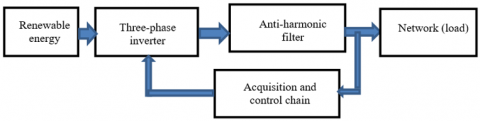Figure 1. Block diagram of island production

2.1 Three-phase inverter modelling

The modelling of the three-phase inverter here consists of determining the output voltage equations as a function of the DC voltage and the switching states of the electronic switches. Figure 2 shows a schematic diagram of a three-phase inverter.

## 2.png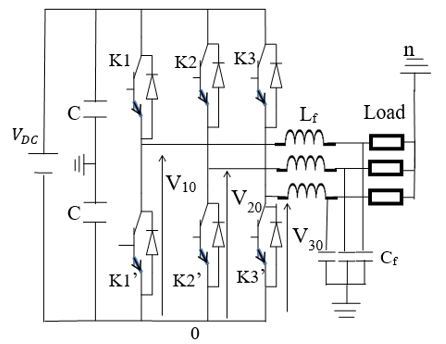Figure 2. Diagram of the three-phase inverter

At any time,

$\left\{\begin{array}{l}\mathrm{V}_{12}=\mathrm{V}_{10}-\mathrm{V}_{20} \\ \mathrm{~V}_{23}=\mathrm{V}_{20}-\mathrm{V}_{30} \\ \mathrm{~V}_{31}=\mathrm{V}_{30}-\mathrm{V}_{10}\end{array}\right.$    (1)

where, $V_{i 0}$ represents the difference in potential between phase i and point o. And $V_{i j}$ represents the difference in potential between phase i and phase j. With i,j=1, 2, 3.

Considering 'n' the neutral point of the alternating load, and if in addition, the network is balanced, we can write :

$\mathrm{V}_{1 \mathrm{n}}+\mathrm{V}_{2 \mathrm{n}}+\mathrm{V}_{3 \mathrm{n}}=0$    (2)

or,

$\left\{\begin{array}{l}\mathrm{V}_{1 \mathrm{n}}=\frac{1}{3}\left(\mathrm{~V}_{12}-\mathrm{V}_{31}\right) \\ \mathrm{V}_{2 \mathrm{n}}=\frac{1}{3}\left(\mathrm{~V}_{23}-\mathrm{V}_{12}\right) \\ \mathrm{V}_{3 \mathrm{n}}=\frac{1}{3}\left(\mathrm{~V}_{31}-\mathrm{V}_{23}\right)\end{array}\right.$    (3)

Then (1) in (2) gives (4):

$\left\{\begin{array}{l}\mathrm{V}_{1 \mathrm{n}}=\frac{1}{3}\left(2 \mathrm{~V}_{10}-\mathrm{V}_{20}-\mathrm{V}_{30}\right) \\ \mathrm{V}_{2 \mathrm{n}}=\frac{1}{3}\left(-\mathrm{V}_{10}+2 \mathrm{~V}_{20}-\mathrm{V}_{30}\right) \\ \mathrm{V}_{3 \mathrm{n}}=\frac{1}{3}\left(\mathrm{~V}_{10}-\mathrm{V}_{20}+2 \mathrm{~V}_{30}\right)\end{array}\right.$    (4)

where: $V_{\text {in }}$ represents the difference in potential between phase i and neutral. With i=1, 2, 3. Let $d_{i}$ be the state of the switch $K_{i}$.

If $d_{i}=1$, then the upper switch is closed and the lower switch is open.

If $d_{i}=0$, then the upper switch is open and the lower switch is closed.

We can therefore establish a relationship between the voltage $V_{i 0}$ and control de-vices $d_{i}$.

$\mathrm{V}_{\mathrm{i} 1}=\mathrm{V}_{\mathrm{DC}}\left(\mathrm{d}_{\mathrm{i}}-\frac{1}{2}\right)$    (5)

Then by combining Eq. (5) and Eq. (4) we obtain:

$\left[\begin{array}{c}\mathrm{V}_{1 \mathrm{n}} \\ \mathrm{V}_{2 \mathrm{n}} \\ \mathrm{V}_{3 \mathrm{n}}\end{array}\right]=\frac{\mathrm{V}_{\mathrm{DC}}}{3}\left[\begin{array}{ccc}2 & -1 & -1 \\ -1 & 2 & -1 \\ -1 & -1 & 2\end{array}\right]\left[\begin{array}{l}\mathrm{d}_{1} \\ \mathrm{~d}_{2} \\ \mathrm{~d}_{3}\end{array}\right]$    (6)

We can therefore build the voltages of $V_{1 n}$, $V_{2 n}$ and $V_{3 n}$ by acting on controls $\mathrm{d}_{\mathrm{i}}$. And these controls can be carried out by our DCM.

2.2 Modelling of the acquisition and control chain of the three-phase inverter

This modelling consists in establishing the interdependence relationships between the electrical quantities, from the acquisition of electrical quantities to the control of electronic switches.

2.2.1 Modelling of the acquisition chain

This modelling is based on Fortescue's principle, which allows us to establish the relations between the network magnitudes (voltage and intensity) and the direct, inverse and homopolar sequences: these relations are as follows [5, 13];

$\left\{\begin{array}{l}{\left[\begin{array}{c}\mathrm{Y}_{\mathrm{A}, \mathrm{d}} \\ \mathrm{Y}_{\mathrm{B}, \mathrm{d}} \\ \mathrm{Y}_{\mathrm{C}, \mathrm{d}}\end{array}\right]=\left[\mathrm{M}_{\mathrm{d}}\right]\left[\begin{array}{c}\mathrm{Y}_{\mathrm{A}} \\ \mathrm{Y}_{\mathrm{B}} \\ \mathrm{Y}_{\mathrm{C}}\end{array}\right]} \\ {\left[\begin{array}{c}\mathrm{Y}_{\mathrm{A}, \mathrm{i}} \\ \mathrm{Y}_{\mathrm{B}, \mathrm{i}} \\ \mathrm{Y}_{\mathrm{C}, \mathrm{i}}\end{array}\right]=\left[\mathrm{M}_{\mathrm{i}}\right]\left[\begin{array}{c}\mathrm{Y}_{\mathrm{A}} \\ \mathrm{Y}_{\mathrm{B}} \\ \mathrm{Y}_{\mathrm{C}}\end{array}\right]} \\ {\left[\begin{array}{c}\mathrm{Y}_{\mathrm{A}, \mathrm{h}} \\ \mathrm{Y}_{\mathrm{B}, \mathrm{h}} \\ \mathrm{Y}_{\mathrm{C}, \mathrm{h}}\end{array}\right]=\left[\mathrm{M}_{\mathrm{h}}\right]\left[\begin{array}{c}\mathrm{Y}_{\mathrm{A}} \\ \mathrm{Y}_{\mathrm{B}} \\ \mathrm{Y}_{\mathrm{C}}\end{array}\right]}\end{array}\right.$    (7)

where, $Y_{A, B, C}$ is the voltage or current vector and M is the Fortescue matrix.

$\left\{\begin{array}{l}\mathrm{M}_{\mathrm{d}}=\frac{1}{3}\left[\begin{array}{ccc}1 & \mathrm{a} & \mathrm{a}^{2} \\ \mathrm{a}^{2} & 1 & \mathrm{a} \\ \mathrm{a} & \mathrm{a}^{2} & 1\end{array}\right] \\ \mathrm{M}_{\mathrm{i}}=\frac{1}{3}\left[\begin{array}{ccc}1 & \mathrm{a}^{2} & \mathrm{a} \\ \mathrm{a} & 1 & \mathrm{a}^{2} \\ \mathrm{a}^{2} & \mathrm{a} & 1\end{array}\right] \\ \mathrm{M}_{\mathrm{h}}=\frac{1}{3}\left[\begin{array}{lll}1 & 1 & 1 \\ 1 & 1 & 1 \\ 1 & 1 & 1\end{array}\right]\end{array}\right.$    (8)

where, $M_{d}$, $M_{i}$ and $M_{h}$ represent respectively the Fortescue matrices of the direct, inverse and homopolar chains.

The real parts of these matrices are extracted by the $R_{e}$ blocks in order to transform them into a Park landmark. The direct and inverse chains being three-phase, and the components of these chains being offset by from each other, they can be easily processed in Park's continuous marker. But the zero sequence chain appears as a disturbance in Park's marker, because all its components are in phase. Its 1200 components must also be out of phase with each other so that they can be easily processed in this benchmark. Thus, the matrice $M_{h}^{*}$ allows us to shift these components.

$\mathrm{M}_{\mathrm{h}}^{*}=\frac{1}{3}\left[\begin{array}{rrr}1 & 1 & 1 \\ \mathrm{a} & \mathrm{a} & \mathrm{a} \\ \mathrm{a}^{2} & \mathrm{a}^{2} & \mathrm{a}^{2}\end{array}\right]$    (9)

2.2.2 Calculator modelling

The calculator (Ca), illustrated in Figure 9, will allow us to regulate the symmetrical components. Its modelling takes into account the filter placed at the output of the inverter, all this in a Park reference. Figure 3 represents this harmonic filter.

## 3.png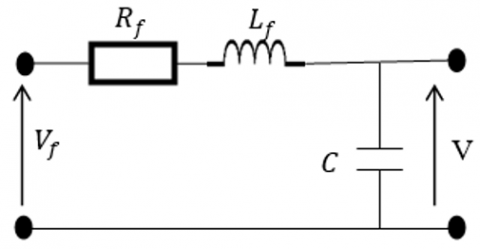Figure 3. Filter diagram

In the Park frame, this filter is modeled as follows [14, 15]:

$\left\{\begin{array}{l}\mathrm{v}_{\mathrm{df}}=\mathrm{R}_{\mathrm{f}} \mathrm{i}_{\mathrm{d}}+\mathrm{L}_{\mathrm{f}} \frac{\mathrm{di}_{\mathrm{d}}}{\mathrm{dt}}-\omega \mathrm{i}_{\mathrm{q}}+\mathrm{v}_{\mathrm{d}} \\ \mathrm{v}_{\mathrm{qf}}=\mathrm{R}_{\mathrm{f}} \mathrm{i}_{\mathrm{q}}+\mathrm{L}_{\mathrm{f}} \frac{\mathrm{di}_{\mathrm{q}}}{\mathrm{dt}}+\omega \mathrm{i}_{\mathrm{d}}+\mathrm{v}_{\mathrm{q}}\end{array}\right.$    (10)

where $V_{d f}$, and $V_{q f}$ represent the forward and quadrature voltages at the input of the filter, $V_{d}$ and $V_{q}$ represent the forward and quadrature voltages at the output of the filter. $R_{f}$ and $L_{f}$, The resistance and inductance of the filter. $I_{d}$ and $i_{q}$: The direct and quadrature currents flowing through the filter, and ω: The pulsation of the electrical quantities.

The equations of this filter can be written by including the symmetrical components.

For the direct chain we have:

$\left\{\begin{array}{l}\mathrm{v}_{\mathrm{d}, \mathrm{df}}=\mathrm{R}_{\mathrm{f}} \mathrm{i}_{\mathrm{d}, \mathrm{d}}+\mathrm{L}_{\mathrm{f}} \frac{\mathrm{di}_{\mathrm{d}, \mathrm{d}}}{\mathrm{dt}}-\omega \mathrm{i}_{\mathrm{q}, \mathrm{d}}+\mathrm{v}_{\mathrm{d}, \mathrm{d}} \\ \mathrm{v}_{\mathrm{q}, \mathrm{df}}=\mathrm{R}_{\mathrm{f}} \mathrm{i}_{\mathrm{q}, \mathrm{d}}+\mathrm{L}_{\mathrm{f}} \frac{\mathrm{di}_{\mathrm{q}, \mathrm{d}}}{\mathrm{dt}}+\omega \mathrm{i}_{\mathrm{d}, \mathrm{d}}+\mathrm{v}_{\mathrm{q}, \mathrm{d}}\end{array}\right.$    (11)

For the reverse chain we have

$\left\{\begin{array}{l}\mathrm{v}_{\mathrm{d}, \mathrm{if}}=\mathrm{R}_{\mathrm{f}} \mathrm{i}_{\mathrm{d}, \mathrm{i}}+\mathrm{L}_{\mathrm{f}} \frac{\mathrm{di}_{\mathrm{d}, \mathrm{i}}}{\mathrm{dt}}-\omega \mathrm{i}_{\mathrm{q}, \mathrm{i}}+\mathrm{v}_{\mathrm{d}, \mathrm{i}} \\ \mathrm{v}_{\mathrm{q}, \mathrm{if}}=\mathrm{R}_{\mathrm{f}} \mathrm{i}_{\mathrm{q}, \mathrm{i}}+\mathrm{L}_{\mathrm{f}} \frac{\mathrm{di}_{\mathrm{q}, \mathrm{i}}}{\mathrm{dt}}+\omega \mathrm{i}_{\mathrm{d}, \mathrm{i}}+\mathrm{v}_{\mathrm{q}, \mathrm{i}}\end{array}\right.$    (12)

For the homopolar chain we have:

$\left\{\begin{array}{l}\mathrm{v}_{\mathrm{d}, \mathrm{hf}}=\mathrm{R}_{\mathrm{f}} \mathrm{i}_{\mathrm{d}, \mathrm{h}}+\mathrm{L}_{\mathrm{f}} \frac{\mathrm{di}_{\mathrm{d}, \mathrm{h}}}{\mathrm{dt}}+\mathrm{v}_{\mathrm{d}, \mathrm{h}} \\ \mathrm{v}_{\mathrm{q}, \mathrm{hf}}=\mathrm{R}_{\mathrm{f}} \mathrm{i}_{\mathrm{q}, \mathrm{h}}+\mathrm{L}_{\mathrm{f}} \frac{\mathrm{di}_{\mathrm{q}, \mathrm{h}}}{\mathrm{dt}}+\mathrm{v}_{\mathrm{q}, \mathrm{h}}\end{array}\right.$    (13)

These equations also allow us to synthesize PI regulators.

Thus, our calculator will compare the voltages $V_{d}$ and $V_{q}$ of each chain to the setpoint voltages $V^{*}{ }_{d}$ and $V^{*} q$ of the same chain, and the same reasoning is applied to the currents. This strategy is illustrated in Figure 9.

2.2.3 Modelling of the duty cycle modulator (DCM)

Principle of operation. The DCM is a relaxation oscillator whose principle is derived from that of a tantalum vase. It works by charging and discharging a capacitor [1, 16]. To this end, it provides double feedback as shown in Figure 4.

## 4.png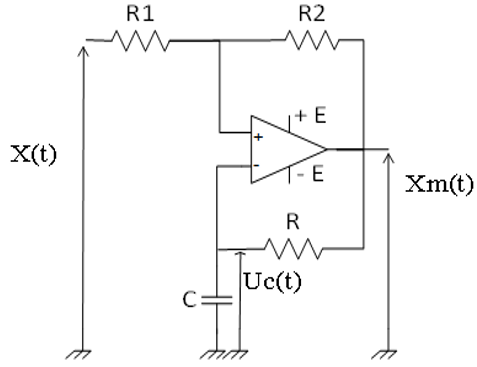Figure 4. Diagram of the DCM

Analysis and equations. Expressions can be expanded as follows :

$\left\{\begin{array}{l}\mathrm{e}^{+}=\frac{\frac{\mathrm{x}}{\mathrm{R}_{1}}+\frac{\mathrm{x}_{\mathrm{m}}}{\mathrm{R}_{2}}}{\frac{1}{\mathrm{R}_{1}}+\frac{1}{\mathrm{R}_{2}}}=\frac{\mathrm{R}_{2} \mathrm{x}+\mathrm{R}_{1} \mathrm{x}_{\mathrm{m}}}{\mathrm{R}_{1}+\mathrm{R}_{2}} \\ \mathrm{e}^{-}=\mathrm{U}_{\mathrm{C}}\end{array}\right.$    (14)

These equations can also be summarized as follow:

$\left\{\begin{array}{l}\mathrm{e}^{+}(\mathrm{t})=\beta \mathrm{x}(\mathrm{t})+\alpha \mathrm{x}_{\mathrm{m}}(\mathrm{t}) \\ \varepsilon(\mathrm{t})=\mathrm{e}^{+}-\mathrm{e}^{-} \\ \mathrm{x}_{\mathrm{m}}(\mathrm{t})=\operatorname{Esign}(\varepsilon(\mathrm{t})) \\ \frac{\mathrm{du}_{\mathrm{c}}(\mathrm{t})}{\mathrm{dt}}=\frac{1}{\mathrm{R}_{1}} \mathrm{u}_{\mathrm{c}}(\mathrm{t})+\frac{1}{\tau} \mathrm{x}_{\mathrm{m}}(\mathrm{t})\end{array}\right.$    (15)

where,

$\alpha=\frac{\mathrm{R}_{1}}{\mathrm{R}_{1}+\mathrm{R}_{2}}, \beta=\frac{\mathrm{R}_{2}}{\mathrm{R}_{1}+\mathrm{R}_{2}}$ and $\tau=\mathrm{RC}$    (16)

Our DCM is therefore characterized by :

Its period $T(x, \alpha)_{m}$

$\mathrm{T}(\mathrm{x}, \alpha)_{\mathrm{m}}=\tau \log \left[\frac{(\beta \mathrm{x})^{2}-((1-\alpha) \mathrm{E})^{2}}{(\beta \mathrm{x})^{2}-((\alpha-1) \mathrm{E})^{2}}\right]$    (17)

Its duty cycle $R_{m}$ .

$\mathrm{R}_{\mathrm{m}}=\frac{\mathrm{T}_{\mathrm{on}}(\mathrm{x}, \alpha)}{\mathrm{T}(\mathrm{x}, \alpha)_{\mathrm{m}}}$    (18)

$\mathrm{R}_{\mathrm{m}}=\frac{\log \left[\frac{(1-\alpha) \mathrm{x}-((1+\alpha) \mathrm{E}}{(1-\alpha) \mathrm{x}-((\alpha-1) \mathrm{E}}\right]}{\log \left[\frac{(\beta \mathrm{x})^{2}-((1-\alpha) \mathrm{E})^{2}}{(\beta \mathrm{x})^{2}-((\alpha-1) \mathrm{E})^{2}}\right]}$    (19)

In linear form, Eq. (19) can be re-written as follows :

$\widetilde{\mathrm{R}}_{\mathrm{m}}=\frac{\alpha \mathrm{x}}{\mathrm{E}(1+\alpha) \log \left(\frac{1+\alpha}{1-\alpha}\right)}+\frac{1}{2}$    (20)

Figure 5 shows the variations of $R_{m}$ and $\widetilde{R}_{m}$ given by Eqns. (19) and (20), as a function of the input voltage x(t). With α=0.6 and E=15 V.

## 5.png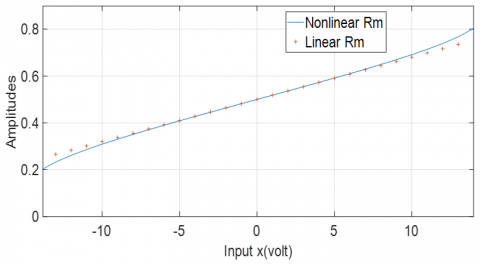Figure 5. Variation of the duty cycle depending voltage

The furnace series of its output signal $X_{k}(t, x, \alpha)$ :

$\mathrm{X}_{\mathrm{k}(\mathrm{t}, \mathrm{x}, \alpha)}=\mathrm{C}_{0}(\mathrm{x})+\sum_{\mathrm{n}=1}^{\infty} \mathrm{C}_{\mathrm{n}}(\mathrm{x}) \cos \left(\frac{2 \mathrm{Int}}{\mathrm{T}_{\mathrm{m}}}\right)$    (21)

where,

$\left\{\begin{array}{l}\mathrm{C}_{0}(\mathrm{x})=\left(2 \mathrm{R}_{\mathrm{m}}(\mathrm{x}, \alpha)-1\right) \mathrm{E} \\ \mathrm{C}_{\mathrm{n}}(\mathrm{x})=\left(\frac{4 \mathrm{E}}{\Pi}\right) \frac{\sin \left(\mathrm{n} \Pi \mathrm{R}_{\mathrm{m}}(\mathrm{x}, \alpha)\right.}{\mathrm{n}}, \mathrm{n} \geq 1\end{array}\right.$    (22)

Oscillating frequency $F(x, \alpha)_{m}$ :

$\mathrm{F}(\mathrm{x}, \alpha)_{\mathrm{m}}=\frac{1}{\operatorname{RC} \cdot \log \left[\frac{(\beta \mathrm{x})^{2}-((1-\alpha) \mathrm{E})^{2}}{(\beta \mathrm{x})^{2}-((\alpha-1) \mathrm{E})^{2}}\right]}$    (23)

for x(t)=0, we have,

$\mathrm{F}(0, \alpha)_{\mathrm{m}}=\frac{1}{2 \mathrm{RC} . \log \left[\frac{(1-\alpha)}{(\alpha-1)}\right]}$    (24)

Figures 6 and 7 show the frequency and pulse variations as a function of the sinusoidal input signal. With $R=R_{1}=10 \mathrm{~K} \Omega$, $R_{2}=8.2 \mathrm{~K} \Omega$, $C=0.8096 n F$, $E=15 \mathrm{~V}$ et x(t) differs from -10 V to 10 V.

## 6.pngFigure 6. Curve of changes in frequency depending on the input voltage

## 7.png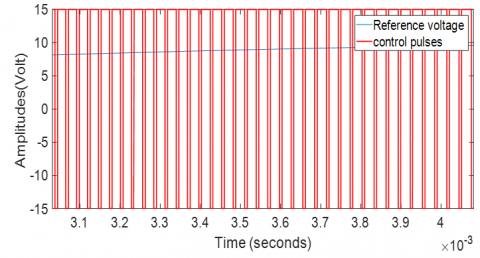Figure 7. Reference voltage and control pulse curve

We can see that the frequency and therefore the duty cycle of the DCM impulses, varies with the input signal x(t), which is an advantage for this modulator.

We can thus summarize the blocks of the acquisition and control chain in Figure 8 below:

## 8.png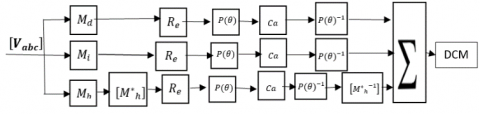Figure 8. Block diagram of the acquisition and control chain

The blocks of each chain are then processed sequentially, starting with the Fortescue transformations ($\boldsymbol{M}_{d}$, $\boldsymbol{M}_{\boldsymbol{i}}$, $\boldsymbol{M}_{\boldsymbol{h}}$). Then the real part of each chain is extracted using the $\boldsymbol{R}_{\boldsymbol{e}}$ blocks. This real part is further transformed in a Park frame of reference in order to be regulated by the Ca calculator block. Finally, the results from the Ca block undergo inverse Park operations, allowing us to restore the reference signals for the MRC modulator block. Finally, the blocks $\boldsymbol{M}^{*}{ }_{\boldsymbol{h}}$ and $\boldsymbol{M}_{h}^{*}{ }^{-1}$ allow us to process the homopolar component in Park's reference frame, without it appearing as a disturbance.

Each chain must be processed at the same time, but independently, hence the parallel processing of chains. The completeness diagram of our strategy is given in Figure 9.

## 9.png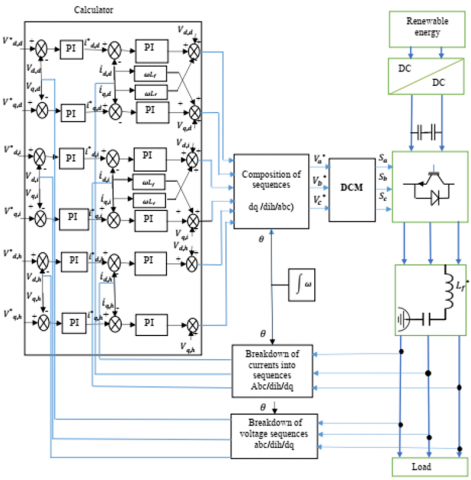Figure 9. Diagram of the three-phase inverter with DCM control

3. Simulations and Analysis of Results

Virtual simulations are performed on the MATLAB Simulink environment to compare the SPWM and DCM control modes, and to analyse the control and regulation of the symmetrical components as well as the behaviour of the inverter in the face of variations in balanced and unbalanced loads.

3.1 Case 1: Comparison of DCM control versus SPWM

The goal here is to choose a modulator which has a reduced THD. because the THD reduction makes it possible to reduce the size of the harmonic filter placed at the output of the inverter. This comparison will be made at the same modulation frequency ($f_{P W M}=f_{D C M}=50 \mathrm{KHz}$), and at the same values of the input voltage (x). and the frequency of the reference voltage (SPWM) is $f_{m}=50 \mathrm{~Hz}$. this simulation exploits Eqns. (21), (22) and (23). Figure 10 shows us the frequency variations of the SPWM and DCM modulators and their THD.

We notice that at x(t)=0 V, the SPWM and DCM modulator have the same oscillation frequency of 50 KHz and the same THD of 147.97%, as shown in Figure 10-a. And at $x=\pm 10 \mathrm{~V}$, the DCM produces a THD of 248.96, while that of the SPWM is 715.74, i.e. 2.7419 times greater than that of DCM. This is due to the fact that, the frequency of the DCM modulator is variable, and that of the SPWM is fixed.

3.2 Case 2: Control of the regulation of symmetrical components

Here, we are testing the inverter's ability to maintain its output voltage constant and balanced in the face of balanced and unbalanced loads. The main features of this inverter are its power of 160 kW, its DC voltage of 700 V and its AC voltages of 230 V (single voltage) and 400 V (compound voltage).

3.2.1 Inverter against variations in Balanced Loads

Simulations are made for power (load) variations, as shown in the following Table 1.

At t=0.15 s, we can see that the load power increases from 80 kW to 160 kW, thus the load current increases from 115 A to 230° (Figure 11-b), and the voltages of the direct chain (Figure 11-c and 11-d), the inverse chain (Figure 11-e and 11-f), the homopolar chain (Figure 11-g and 11-h) and load voltage (Figure 11-a), undergo the slight transient oscillations. Then at t=0.157, these voltages are regulated to their set values. These small oscillations are due to the load variation.

## 10.png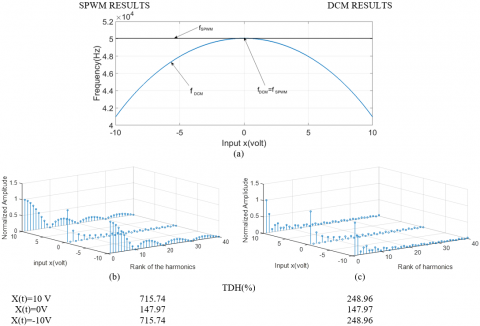Figure 10. Frequency spectrum of the voltage Xm(t) as a function of x(t)

Simulation results

## 11a.png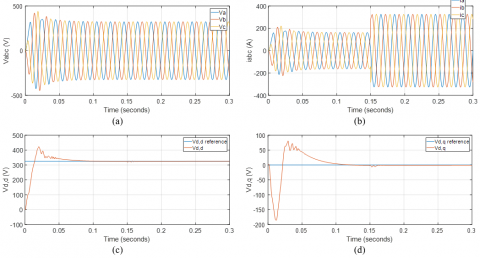## 11b.png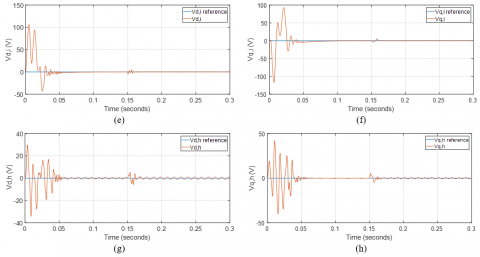Figure 11. Voltage and current curves of the inverter in balanced operation

Table 1. Power variation in balanced mode

 Times (s) [0, 0.15] [0.15, 0.3] Power of Phase a (kW) 160/6 160/3 Power of Phase b (kW) 160/6 160/3 Power of Phase c (kW) 160/6 160/3

3.2.2 Simulation of the inverter in the face of load imbalances

Simulations are made for power (load) variations, as shown in the following Table 2.

From t=0.15 s to t =0.2 s, we see that the power of phase b goes from 53.3333 kW to 0 kW, and the powers of phases a and c remain at 53.3333 kW.  Thus, the current intensity of phase b increases from 230 A to 0 A, and the current intensities of phases a and c remain at 230 A(Figure-12-b). And the voltages of the direct chain (Figures 12-c and 12-d), reverse chain (Figures 12-e and 12-f), homololar chain (Figures 12-g and 12-h), and load voltage (Figure 12-a) remain almost constant and regulated at their set values. From t=0.2s to t=0.25, we observe slight oscillations on the voltages, this is due to the load pick-up of phase b.

Table 2. Power variation in unbalanced mode

 Temps(s) [0, 0.15] [0.15, 0.2] [0.2, 0.3] Power of Phase a (kW) 160/3 0 160/3 Power of Phase b (kW) 160/3 160/3 160/3 Power of Phase c (kW) 160/3 160/3 160/3

Results

## 12a.png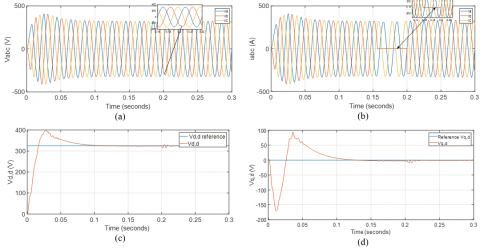## 12b.pngFigure 12. Curves of inverter voltages and currents in unbalanced mode

4. Conclusion

This article was devoted to the presentation of a new control strategy which consists of the duty cycle modulator (DCM), the Park (dq) and Fortescue (dih) transformation, for optimal control of three-phase inverters. The principle of this strategy is to exploit the DCM in order to reduce the THD of three-phase inverters. And also, to exploit the transformation of Fortescue to regulate the symmetrical components (dih) in order to keep the output voltage of the inverter balanced. To test the effectiveness of the strategy, we have compared DCM and SPWM first on their THD by exploiting their Fourier series decomposition. The results of this test, show us that the DCM has a better THD. And secondly, the whole strategy ((DCM-dq-dih) was tested on balanced and unbalanced loads. And the test results show us that the output voltage of the inverter remains constant and balanced even when there is a load imbalance.

In future research works, it would be interesting to transform the virtual reality study into realistic implementation. It would also be nice to use the smart regulators to optimize the results. And finally, it would also be very interesting to optimize the synchronization of the inverters on the interconnected networks by exploiting this strategy.

Nomenclature
 DCM Duty Cycle Modulation PWM Pulse Width Modulation SPWM Sinusoidal Pulse Width Modulation SVPWM Space Vector Pulse Width Modulation Dq Park Components (direct and quadrature) dih Fortescue Components (Direct, reverse and homopolar) M Matrix I current V voltage DC Direct Current THD Total Harmonic Distortion R resistance L inductance C capacitor Ca calculator f frequency Re The real part $V_{d, d}$ direct, direct voltage $V_{q, d}$ quadrature, direct voltage $V_{d, i}$ direct, reverse voltage $V_{q, i}$ quadrature, reverse voltage $V_{d, h}$ direct, homopolar voltage $V_{q, h}$ quadrature, homopolar voltage $i_{d, d}$ direct, direct current $i_{q, d}$ quadrature, direct current idi direct, reverse current iqi quadrature, reverse current $i_{d, h}$ direct, homopolar current $i_{q, h}$ quadrature, homopolar current Vdf Filter direct voltage Vqf Filter quadrature voltage $M_{d}$ Direct Fortescue matrix Mi Reverse Fortescue matrix $M_{h}$ Homopolar Fortescue matrix $R_{m}$ Nonlinear Duty Cycle Modulator $\tilde{R}_{m}$ Linear Duty Cycle Modulator $x(t)$ Signal to modulate xm Signal modulated by the DCM τ The time constant β Constant without dimension a Constant without dimension $T(x, \alpha)$ DCM period $F(x, \alpha)$ DCM frequency
References

 Biyobo, A.O., Nneme, L.N., Mbihi, J., Pauné, F., Moffo, B.L. (2020). Étude expérimentale d’un nouveau modèle d’onduleur solaire monophasé à modulation en rapport cyclique. Afrique SCIENCE, 16(2): 118-131.

 Taleb, R., Helaimi, M.H., Benyoucef, D., Boudjema, Z. (2015). Commande par MLI pré-calculée d’un onduleur asymétrique. Application à la commande vectorielle d’une MAS de forte puissance. Mémoire, Université De Chlef.

 Benali, F.H., Azzouz, F. (2016). L'influence de la variation de l'indice de modulation et du taux de la fréquence sur les performances de sortie de l'onduleur multi niveaux en pont H. 9th International Conference on Electrical Engineering and first workshop on robotics and controls ICEE.

 Taleb, R., Ziane, A.B.B., Bessaad, T., Derrouazin, A. (2014). Commande par la stratégie d’élimination d’harmoniques d’un onduleur asymétrique à onze niveaux. 3rd International Seminar on New and Renewable Energies, SIENR (Vol. 14).

 Habib, B., Rachid, T., Fayçal, C. (2016). Etude comparative de deux stratégie de commande DTC neuronale d’une MAS alimentée par onduleur à sept niveaux. Acta Electrotehnica, 57(5): 570-578.

 Samir, A., Abdellah, A. (2013). Etude comparative de deux stratégies PWM un onduleur multi-niveaux. Doctoral dissertation, Université Mouloud Mammeri.

 Khaled, S., Mourad, S.M. (2015). Commande MLI d’un onduleur multi-niveaux. Doctoral dissertation, Université Mouloud Mammeri.

 Chaoui, A. (2010). Filtrage actif triphase pour charges non linéaires. Thèse PhD, Poitiers.

 Kerrouche, F., Tazerart, F., Taib, N. (2020). Novel topology of a multilevel inverter dedicated to electric traction drive. European Journal of Electrical Engineering, 22(3): 255-263. https://doi.org/10.18280/ejee.220306

 Biyobo, A.O., Nneme, L.N., Mbihi, J.E.A.N. (2018). A novel sine duty-cycle modulation control scheme for photovoltaic single-phase power inverters. WSEAS Transactions on circuits and Systems, 17: 107-113.

 Etouke, P.O., Nneme, L.N., Mbihi, J. (2020). An optimal control scheme for a class of duty-cycle modulation buck choppers: Analog design and virtual simulation. Journal of Electrical Engineering, Electronics, Control and Computer Science, 6(1): 13-20.

 Nnem, L.N., Lonla, B.M., Sonfack, G.B., Mbihi, J. (2018). Review of a multipurpose duty-cycle modulation technology in electrical and electronics engineering. Journal of Electrical Engineering, Electronics, Control and Computer Science, 4(2): 9-18.

 Vechiu, I. (2005). Modélisation et analyse de l'intégration des énergies renouvelables dans un réseau autonome. Doctoral dissertation, Université du Havre.

 Hocini, N., Medjoudj, R., Tazamouchet, E. (2016). Sur la maitrise de l'insertion des ressources renouvelables aux réseaux électriques. Doctoral dissertation, Université Abderrahmane Mira-Bejaia.

 Le Moigne, P., Delarue, P., Fernandez, S. (2014). Modulation par hystérésis de courant à mémoire d'état d'un onduleur triphasé deux niveaux-comportement électrique. Symposium de Génie Électrique 2014.

 Otam, S. (2016). Conception et Simulation d’un Convertisseur A/N multicanaux pour carte FPGA. Master recherche, Universite de Douala.

 Sonfack, G., Mbihi, J., Moffo, B.L. (2017). Optimal duty-cycle modulation scheme for analog-to-digital conversion systems. International Journal of Electronics and Communication Engineering, 11(3): 354-360. https://doi.org/10.5923/j.eee.20180802.01